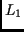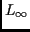Next: Application Areas Up: Distance Fields in Visualization Previous: Distance Fields in Visualization

# Distance Transforms

Brute force distance calculations are very expensive, since for each voxel of the field the distance to the nearest surface point has to be evaluated by inspecting all objects of the scene. Satherley and Jones [SJ01] reported days long calculations on high-end workstations. Approximate techniques have been therefore developed, cumulatively known as distance transforms [RP66], which try to estimate the Euclidean distance in a reasonable time. Their main idea is to replace the global distance computation by a local propagation of distances in a small neighborhood. These approaches require several passes through the data.

Chamfer distance transforms, proposed by Borgefors [Bor86], issue from an assumption that the distance can be computed only from values at neighboring positions plus a mask constant. This approach enables precise computation the city-block (metrics) and chessboard (metrics) distances, but can only approximate the Euclidean distance (metrics). Therefore, more precise albeit slower Vector distance transforms were introduced [Dan80], propagating also position of the nearest surface point. To speed up the computation, the multipass mask propagation was later replaced by region growing [Cui97] and level-set [Set99,BMW98] approaches.

Traditionally, the distance computation issues from a segmented binary data, where the object surface voxel positions are confined to fixed grid point coordinates. However, mostly in volume graphics applications, this proved to be a precision limitation (for example, in offset surface computation [BMW98,SJ01]) and therefore techniques were developed, propagating distances to surfaces defined with subvoxel precision. To compute the field with even higher precision, for each voxel a corresponding nearest surface point is recomputed, utilizing information stored with its already processed neighbors.Next: Application Areas Up: Distance Fields in Visualization Previous: Distance Fields in Visualization
2002-04-09